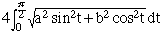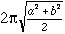I am planning to build a coffe table with an ellipse of 24x36 for the top. I wish to decorate the edge and need to know the lenght of the perimeter for lay out purposes. Is there an easy way to approximate this figure with out using intergal calculus? SawDust Harry Hi Harry, I am assuming that the 24 and 36 are inches. There is a response to an earlier question that gives the answer aswhere the ellipse has semi-axes of lengths a and b. There is no simple expression for this integral but it can be approximated byYou have a = 36/2 = 18 and b = 24/2 = 12 and hence the perimeter is approximately 96.1 inches. We used a computer program called Mathematica to approximate the integral and got the perimeter to be 95.2 inches. Cheers, Penny Go to Math Central